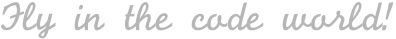| Swift , iOS

## 内容概览

• 前言

• `self``Self`

• `==``===`

• 总结

## `self` 和 `Self`

##### 实例中的 `self`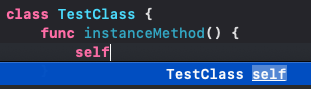##### 类型中的 `self`：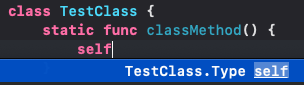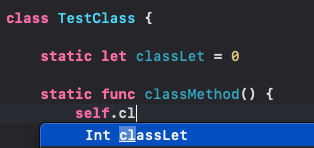##### 实体类型(concrete type)中的 `Self`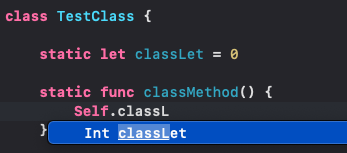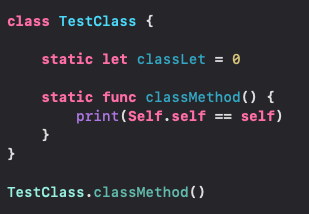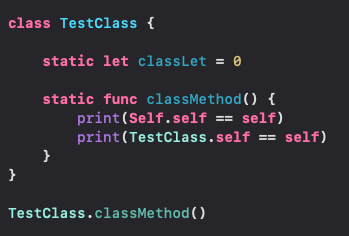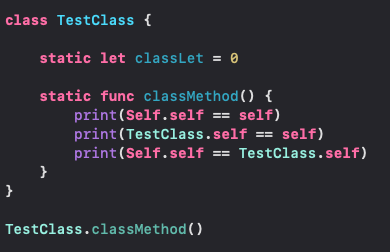``````class TestClass {
static let classLet = 0

let instanceLet = classLet // 不能写成 self.classLet
var instanceVar = classLet

lazy var instanceLazyVar = Self.classLet // 不能写成 self.classLet
}
``````

``````protocol TestProtocol {
func getSelf() -> Self
}
class TestBaseClass: TestProtocol {
func getSelf() -> Self {
return self
}
}
class TestSubclass: TestBaseClass {
override func getSelf() -> Self {
return self
}
}
let base: TestBaseClass = TestBaseClass()
let sub: TestSubclass = TestSubclass()
``````

## `==` 和 `===`

##### `==`
• `Equatable` 协议中定义的方法：`static func == (lhs: Self, rhs: Self) -> Bool`
• 否定形式：`!=`
• 支持自定义比较，规则可以由开发者自行定义比较的规则；

``````class MyType: Equatable {
let id: UUID
let name: String

init(id: UUID, name: String) {
self.id = id
self.name = name
}

static func == (lhs: MyType, rhs: MyType) -> Bool {
// lhs: left-hand side, rhs: right-hand side

// 也可以是： return lhs.id == rhs.id，规则由你来定义
return lhs.id == rhs.id && lhs.name == rhs.name
}
}

// 还可以这样定义：
class MyType: Equatable {
let name: String

init(name: String) {
self.name = name
}

static func == (lhs: MyType, rhs: MyType) -> Bool {
// ObjectIdentifier 不支持值类型
// 也可以这样比较： return lhs === rhs
return ObjectIdentifier(lhs) == ObjectIdentifier(rhs)
}
}
``````

##### `===`
• 比较的是指针，所以只能用于比较引用类型；
• 否定形式：`!==`
• 不支持开发者自定义比较的规则；

``````NSObject() === NSObject()
``````

## 总结

#### 评论区(期待你的留言)